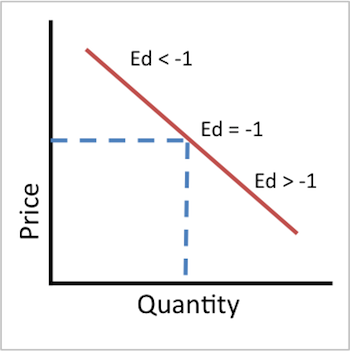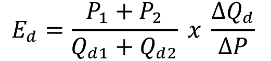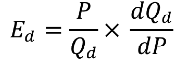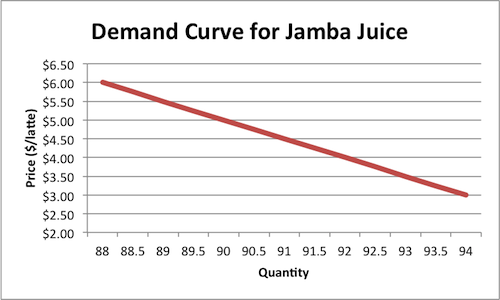# Inelastic Demand: Definition & Examples

Lesson Transcript
Instructor: Kallie Wells

Kallie has a B.S. in Agribusiness and minor in Statistics from California Polytechnic State University, San Luis Obispo and M.S. in Agricultural and Resource Economics from University of California, Davis. She has extensive experience designing and performing economic analysis of wholesale energy markets and investigations of market participant behavior within these markets.

Inelastic demand means that there is little to no change in the quantity demanded by the consumers even if the price of a good or service changes. Learn about the definition of inelastic demand, the different ways to calculate the price elasticity of demand, such as through using the arc price elasticity formula or the price point elasticity formula, and review some examples illustrating how to use these two formulas. Updated: 09/05/2021

## Definition

A demand curve is considered inelastic when it is not very sensitive to price changes. More specifically, a one percent change in price will result in less than a one percent change in quantity demanded, that is, price elasticity between zero and negative one (not inclusive of zero and negative one).

Since demand for most goods is downward sloping, the elasticity of demand will always be negative. Sometimes the negative sign is ignored by taking an absolute value. In that event, an elastic demand will have price elasticity greater than one. In this lesson, the negative sign will be represented. Elasticity of demand is evaluated in terms of percent change in quantity relative to a percent change in price.

Elasticity of demand = % change in quantity demanded / % change in price

It is not to be confused with the slope of the demand curve. The slope of the demand curve remains constant; however, the elasticity of the demand curve changes as you move along. The only curve that has a constant inelastic demand is a vertical demand curve. Any change in price will result in no change in quantity demanded.This graph illustrates elasticity changes along the demand curve. This is for illustration purposes only. To accurately determine at what point a demand curve is inelastic, you will have to calculate the elasticity at varying points on the demand curve.An error occurred trying to load this video.

Try refreshing the page, or contact customer support.

Coming up next: Circular Flow of Economic Activity: The Flow of Goods, Services & Resources

### You're on a roll. Keep up the good work!

Replay
Your next lesson will play in 10 seconds
• 0:00 Definition
• 1:27 Arc Price Elasticity Formula
• 2:31 Price Point Elasticity Formula
• 3:14 Example: Arc Method
• 4:55 Example: Price Point Method
• 6:05 Lesson Summary
Save Save

Want to watch this again later?

Timeline
Autoplay
Autoplay
Speed Speed

## Arc Price Elasticity Formula

Price elasticity of demand can be calculated using the arc or price point method. The arc price formula is Ed equals P1 plus P2 over Qd1 plus Qd2, multiplied by the change in Qd over the change in P, where P1 and P2 are two price points on the demand curve and Qd1 and Qd2 are the quantity demanded given P1 and P2.Therefore, delta Qd = Qd2 - Qd1

delta P = P1 - P2

This method is used primarily when you either don't have a mathematical formula for the demand curve, or you aren't familiar with taking derivatives of equations. The arc method essentially assumes a linear demand curve between the two points when estimating the price elasticity. Therefore, the more curved the demand is between the two points, the more inaccurate the estimate.

## Price Point Elasticity FormulaThe price point elasticity formula is where elasticity of demand equals P over Qd multiplied by d Qd over d P.

P is the price at which you are evaluating the elasticity of demand.

Qd is the quantity demanded at the point you are evaluating elasticity of demand.

dQd/dP is the first derivative of quantity demanded with respect to price.

This is the more accurate method since it uses derivatives to determine the price elasticity at a given point on the demand curve. Calculus allows us to minimize the 'arc' used to estimate elasticity in the arc method, such that it becomes a single point on the demand curve.

## Example: Arc Method

It's summer - let's go to Jamba Juice! This graph shows the demand curve for Jamba Juice in the summer. The demand curve is:

Qd = 100 - 2PUsing the arc method, determine the price elasticity at $4/smoothie. Given the demand equation, first determine the quantity demanded at$4/bottle.

Quantity demanded = 100 - 2(4) = 100 - 8 = 92

(P1, Qd1) = (4, 92)

To unlock this lesson you must be a Study.com Member.

### Register to view this lesson

Are you a student or a teacher?